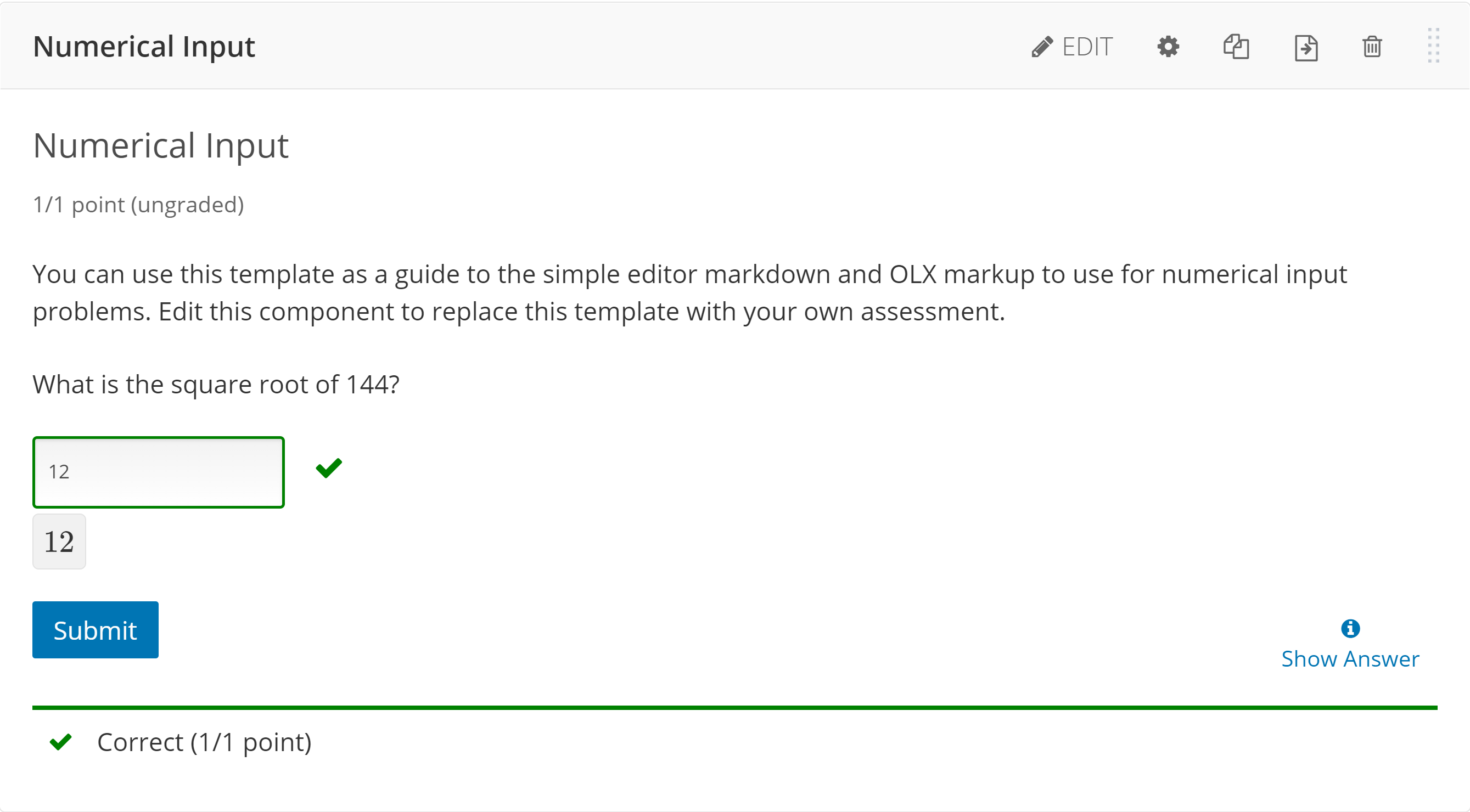# Creating numerical input problems in Open edX

Numerical Input questions, while primarily designed for mathematical calculation problems, can be used in a number of different ways.

From Studio, navigate to the unit of your course where you would like to add a problem, then select the Problem button.You'll now be presented with a list of different problem types. Select Numerical Input.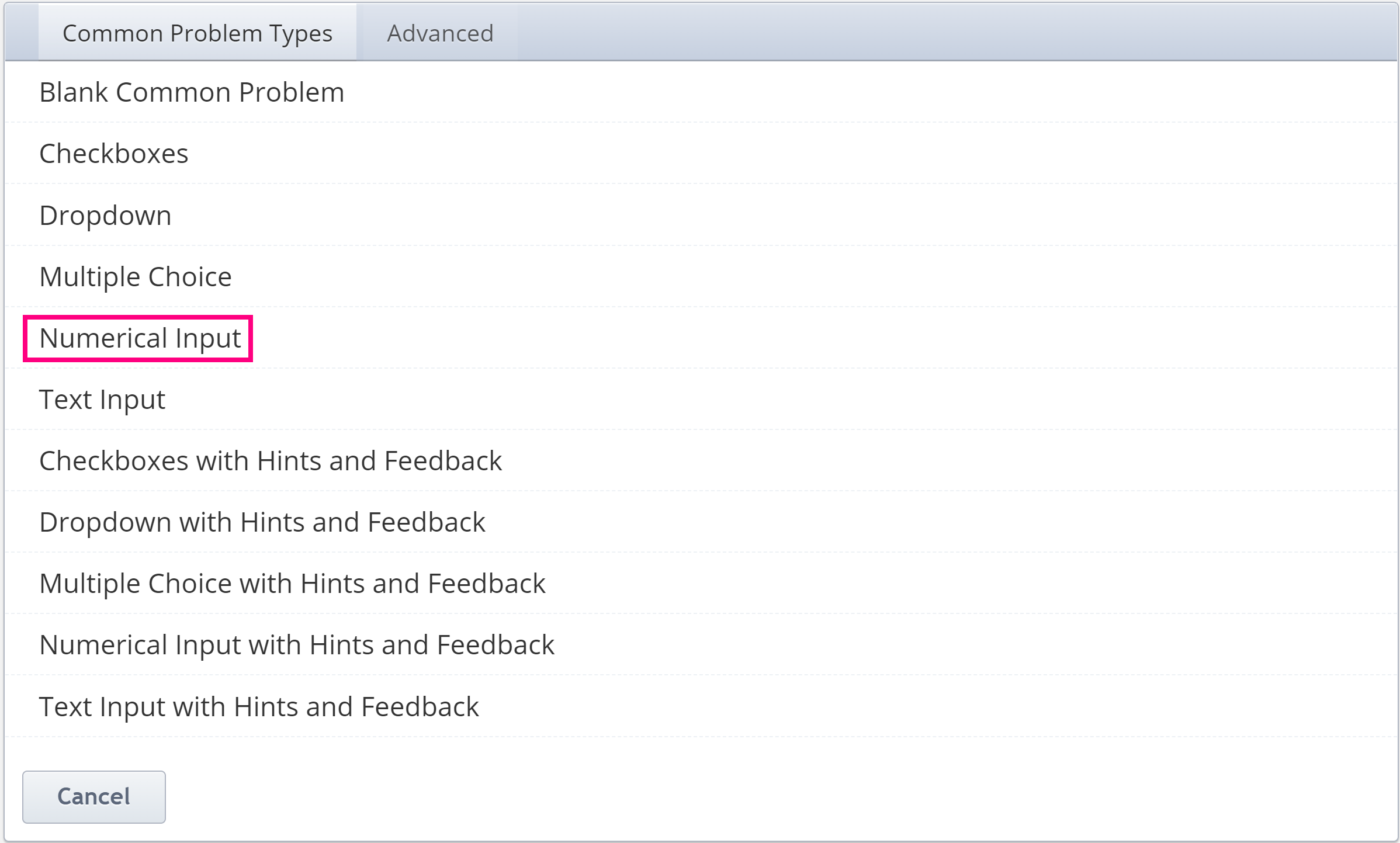You should now see something that looks like the image below. In order to edit your question. Click Edit on the upper right.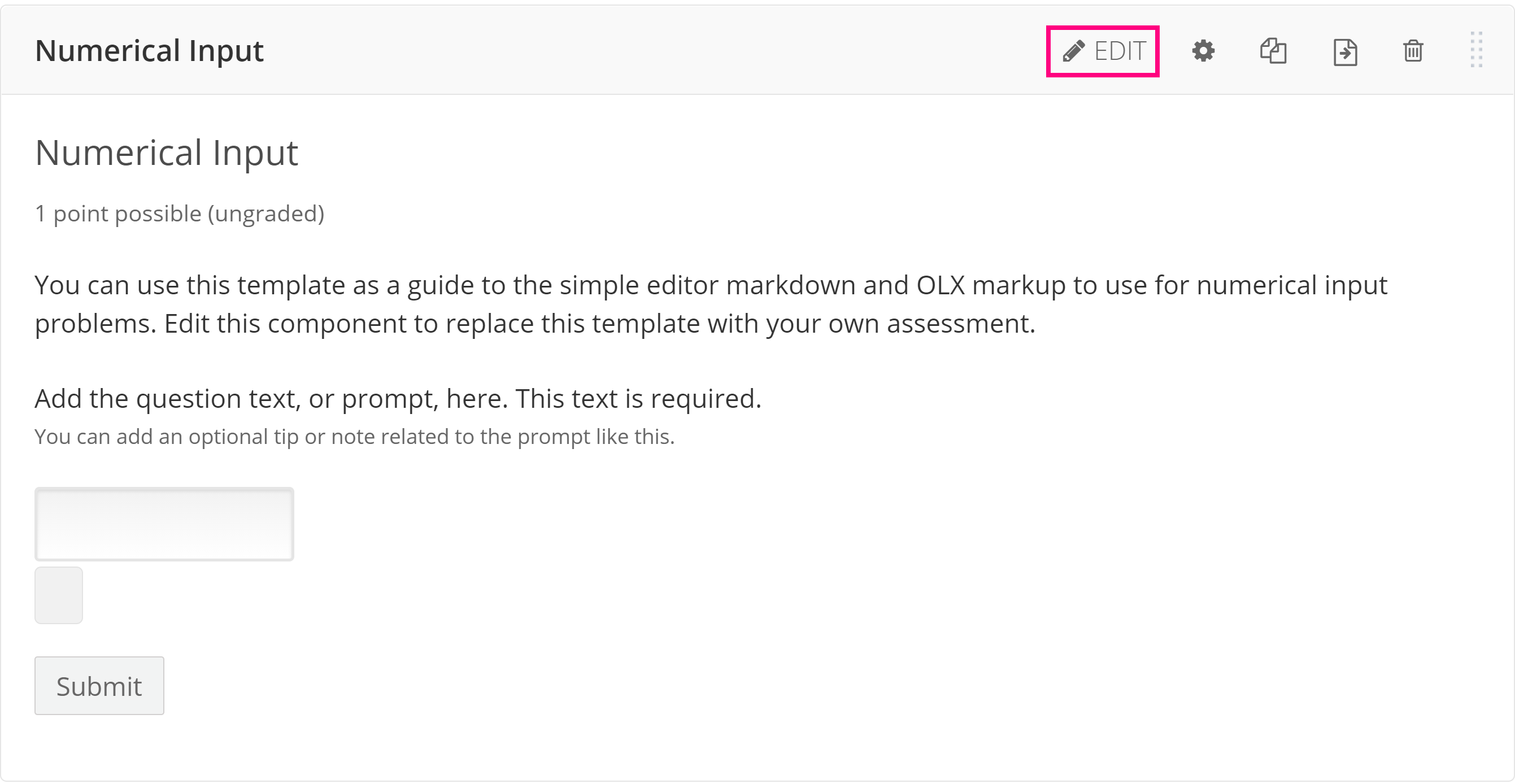This is the Simple Editor where you edit simply. In order to change anything, all you have to do is type, but be mindful of how edX has the problems formatted, and try remain within those boundaries.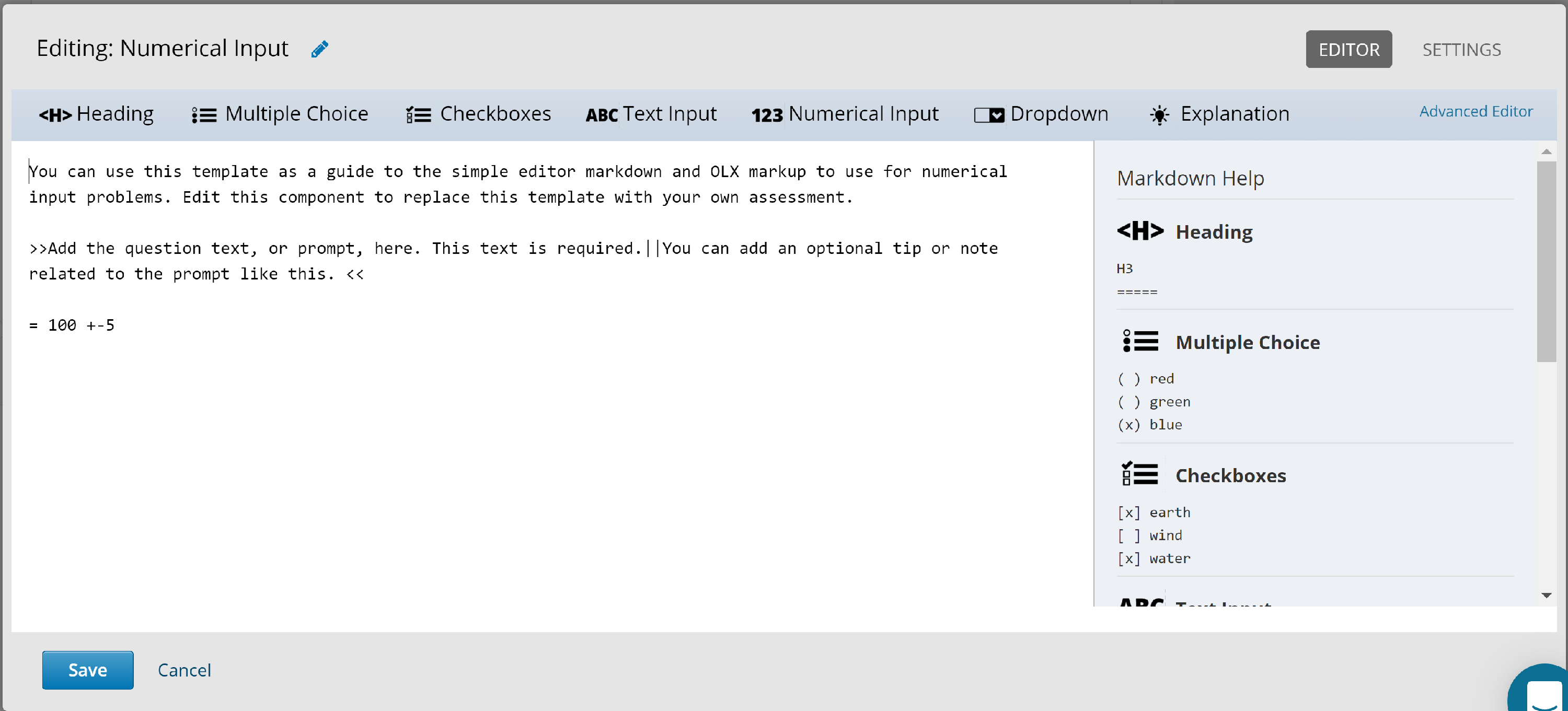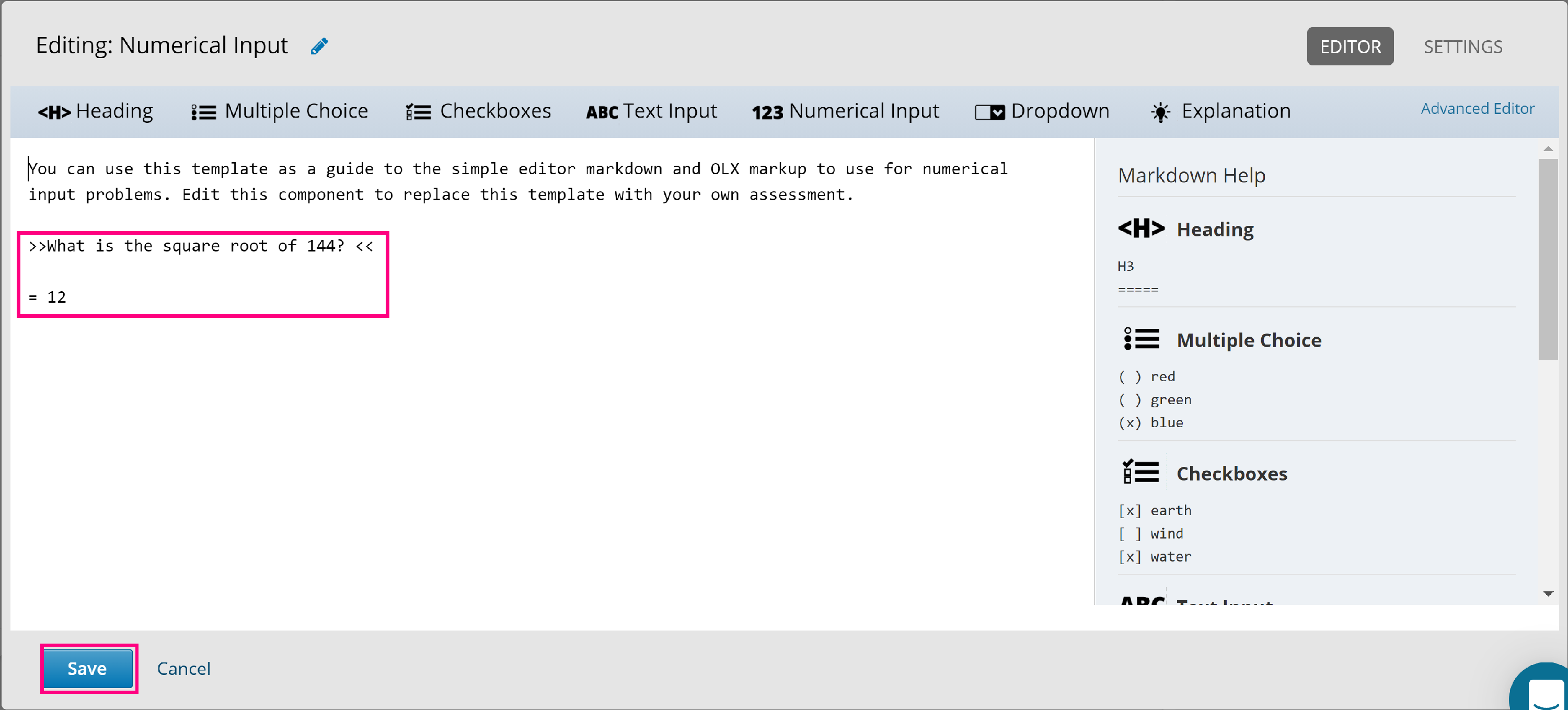Responses for numerical input problems can include integers, fractions, and constants such as  pi and g. Responses can also include text representing common functions, such as square root (sqrt) and log base 2 (log2), as well as trigonometric functions and their inverses, such as sine (sin) and arcsine (arcsin). For these functions, the text that the student enters is converted into mathematical symbols.

When you finish editing your question, be sure to hit Save.